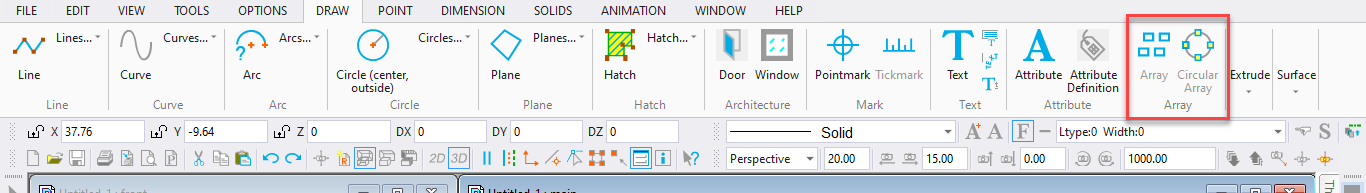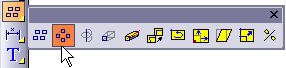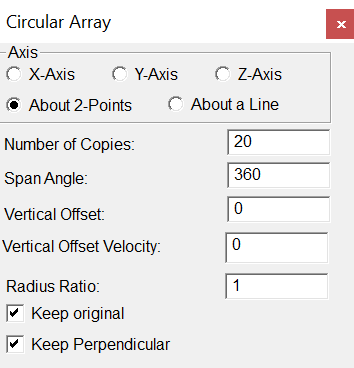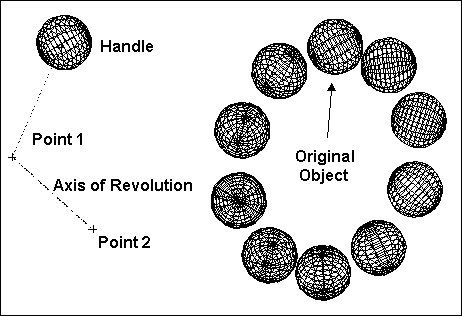# Circular ArrayToolbox Icon:Copies a selected object a specified number of times in a circular pattern.

Point 1: Center of rotation

Point 2: Axis of revolution (Used only with Two-Point Axis mode)

Select the object to copy, then choose the Circular Array command. The Circular Array window appears:Axis: The axis of revolution. You can set the rotation along the X, Y, or Z axis, an axis defined by two points, or one defined by an existing line. Only the About 2Point option requires you to set a second point. With the About a Line option you set a point on an existing line and the command uses that line as the axis of rotation. The other options will use your first point as the location of the axis.

Number of Copies: The total number of copies, including the original.

Span Angle: The angle in which the copies are to be placed. For example, you can use 360° to copy an object in a complete circle, such as the bolts on a wheel. You can use 180° to copy in a semicircle.

Vertical Offset: This is the distance along the axis of revolution between the first copy and the last copy. The offset can be used to copy objects in a spiral. To make a spiral with more than one revolution, use a span angle of more than 360°. For example, to make a spiral with two complete revolutions, you can enter 720° for the span angle. Remember, the number of copies and the offset are the total for the entire operation, not just one revolution.

Vertical Offset Velocity: The ratio between the initial pitch of the offset and the final pitch of the offset. If a value of 5 is used, the final pitch will be 5 times greater than the initial pitch. If a value of 0 or 1 is used, the pitch will remain constant.

Radius Ratio: The distance from the selection handle to the axis, used for the array radius at the beginning of the array. The final radius for the array equals the beginning array radius multiplied by the Radius Ratio. If a value of 1 is used, the radius stays the same throughout the array. If a value greater than 1 is used, the distance from the axis to the duplicated items increases throughout the array. If a value less than 1 is used, the distance from the axis to the duplicated items decreases throughout the array.

Keep Perpendicular: The copies will rotate with the array. If disabled, the copies will still be copied in a circular pattern, but they will maintain the same orientation as the original.

Example: Copy an object 10 times in a circular pattern.

Select the object you want to copy. Next, choose the Circular Array command. Enter the following settings: Number of Copies = 10, Span Angle = 360, Vertical Offset = 0.

Use the About 2 Points option. Set a point a few inches below the object for the center of rotation and another, offset from the first, for the axis of revolution. It may help to think of this as swinging a ball on a string: The selection handle is where the string is connected to the ball, the center of rotation (Point 1) is where your hand holds the string, and the axis of revolution (Point 2) is the direction of your arm.